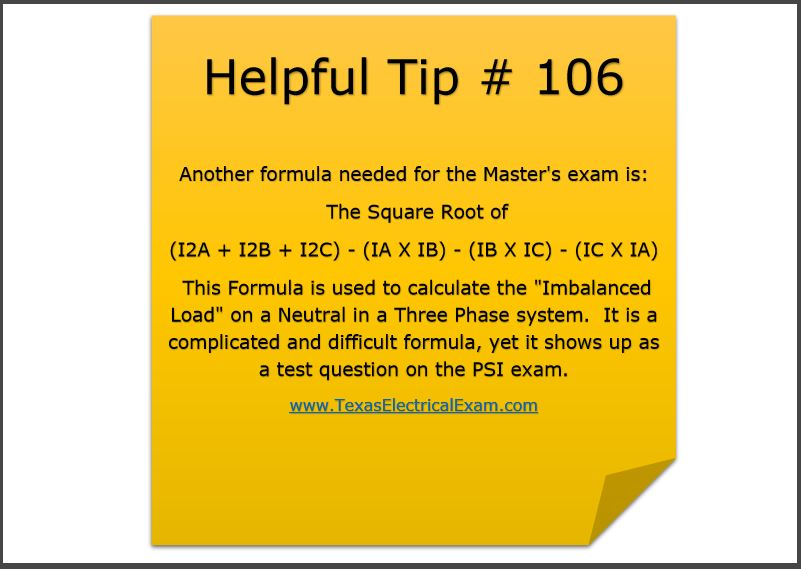# Electrical Exam Prep Tip #106 – Calculate the “Imbalanced Load”Helpful Tip # 106
Another formula needed for the Master's exam is: The Square Root of (I2A + I2B + I2C) - (IA X IB) - (IB X IC) - (IC X IA) This Formula is used to calculate the "Imbalanced Load" on a Neutral in a Three Phase system. It is a complicated and difficult formula, yet it shows up as a test question on the PSI exam.
nd more.

www.TexasElectricalExam.com